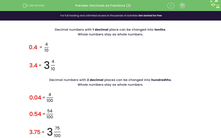# Decimals as Fractions (2)

In this worksheet, students must read and write decimal numbers as fractions or mixed numbers.Key stage:  KS 2

Curriculum topic:   Number: Fractions, Decimals and Percentages

Curriculum subtopic:   Know Decimal Numbers

Popular topics:   Fractions worksheets, Percentages worksheets

Difficulty level:#### Worksheet Overview

Decimal numbers with 1 decimal place can be changed into tenths.

Whole numbers stay as whole numbers.

 0.4 = 4 10

 3.4 = 3 4 10

Decimal numbers with 2 decimal places can be changed into hundredths.

Whole numbers stay as whole numbers.

 0.04 = 4 100

 0.54 = 54 100

 3.75 = 3 75 100

### What is EdPlace?

We're your National Curriculum aligned online education content provider helping each child succeed in English, maths and science from year 1 to GCSE. With an EdPlace account you’ll be able to track and measure progress, helping each child achieve their best. We build confidence and attainment by personalising each child’s learning at a level that suits them.

Get started